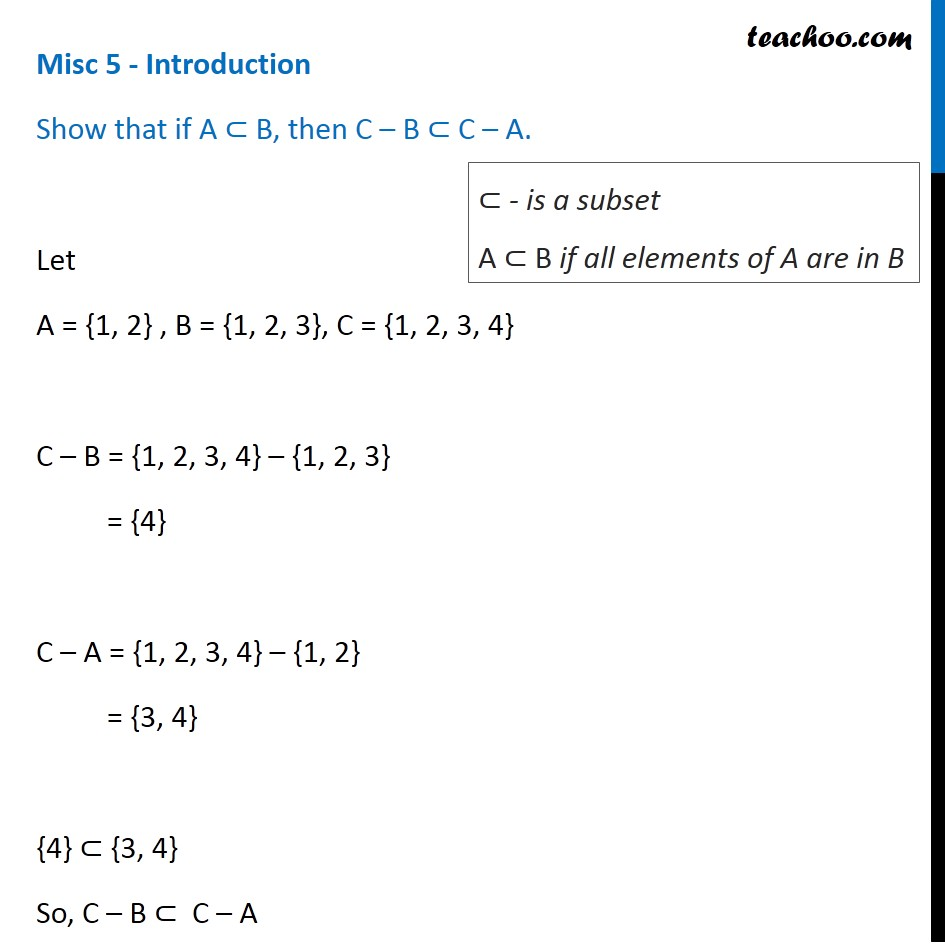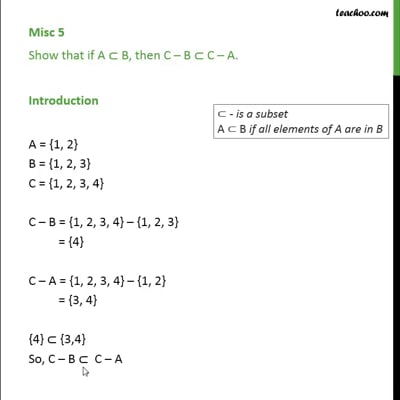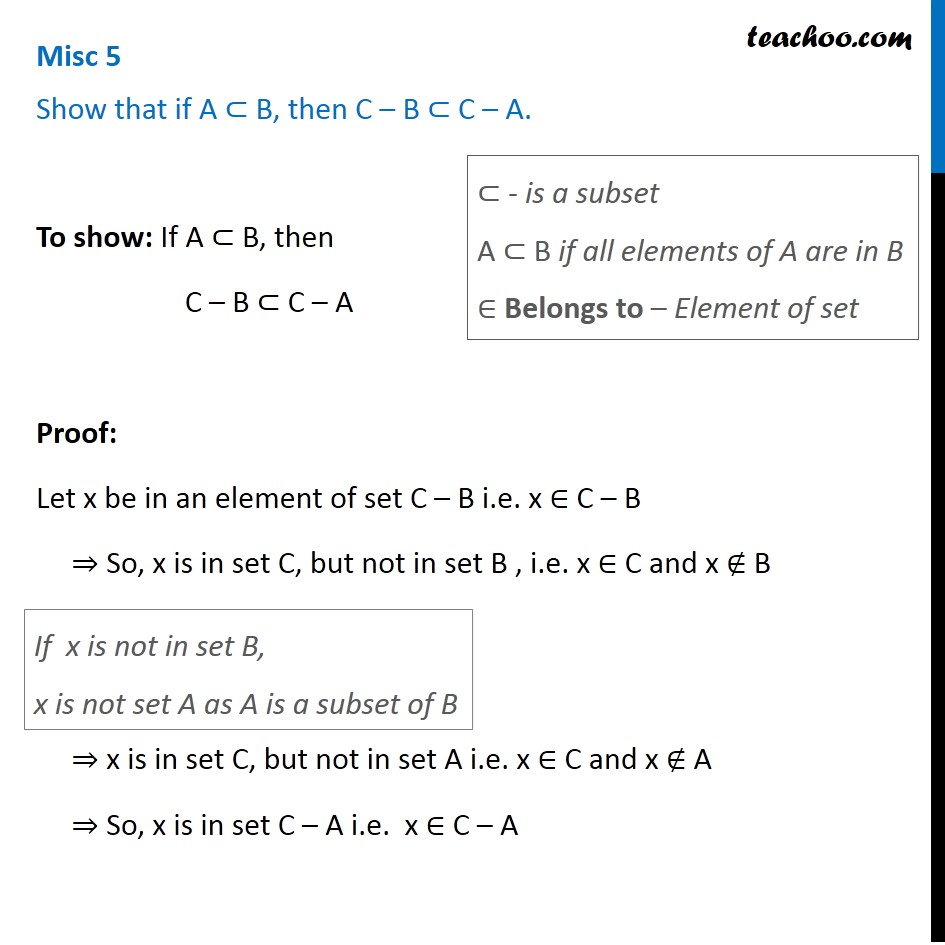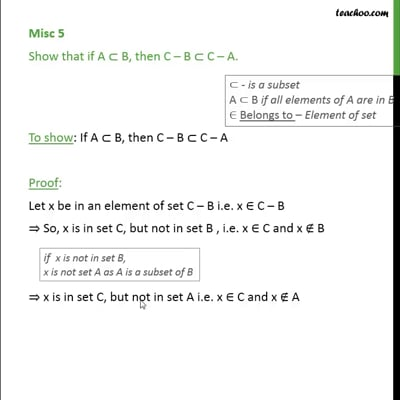Miscellaneous

Chapter 1 Class 11 Sets
Serial order wiseThis video is only available for Teachoo black usersThis video is only available for Teachoo black users

Solve all your doubts with Teachoo Black (new monthly pack available now!)

### Transcript

Misc 5 - Introduction Show that if A ⊂ B, then C – B ⊂ C – A. Let A = {1, 2} , B = {1, 2, 3}, C = {1, 2, 3, 4} C – B = {1, 2, 3, 4} – {1, 2, 3} = {4} C – A = {1, 2, 3, 4} – {1, 2} = {3, 4} {4} ⊂ {3, 4} So, C – B ⊂ C – A ⊂ - is a subset A ⊂ B if all elements of A are in B Misc 5 Show that if A ⊂ B, then C – B ⊂ C – A. To show: If A ⊂ B, then C – B ⊂ C – A Proof: Let x be in an element of set C – B i.e. x ∈ C – B ⇒ So, x is in set C, but not in set B , i.e. x ∈ C and x ∉ B ⇒ x is in set C, but not in set A i.e. x ∈ C and x ∉ A ⇒ So, x is in set C – A i.e. x ∈ C – A If x is not in set B, x is not set A as A is a subset of B ⊂ - is a subset A ⊂ B if all elements of A are in B ∈ Belongs to – Element of set ∴ If x ∈ C – B ,then x ∈ C – A i.e. If an element belongs to the set C – B , it also belongs to the set C – A ⇒ C – B ⊂ C – A Hence proved